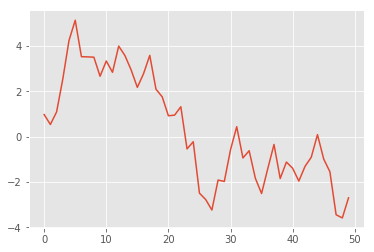# Windy Walk (part 2)

Recap: Previously we constructed a very simple class to emulate the type of environment which is provided by OpenAI. Then we simulated two windy walks and used the result of these walks to produce some plots.

Now we want to produce the same results again but via a different and more interesting route. This time we want a windy walk model that is implemented as an extension to OpenAI.

For this we create a custom python package, we name it gym_drifty_walk.

You can pull the source code of gym_drifty_walk from github .

To install gym_drifty_walk locally do a clone, cd into the gym_drifty_walk directory,  then run python setup.py install.

How the package is created in the first place will be explained in the next post. Let’s look how we use it first.

So first import some packages (the usual suspects … numpy, matplotlib)
In :

Now import the custom environment …

In :

Run the simulation and plot

In :
Out:So this plot, depicting the Position as a function of time of the the first walk, is exactly equal to that of the previous post. That is the way is should be. This  is due to the fact that the same random seed was used, for reproducibility, a standard technique, of course.
So it works!